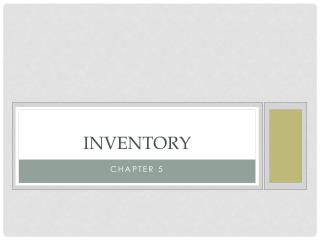DownloadDownload PresentationInventory

# Inventory

Download Presentation## Inventory

- - - - - - - - - - - - - - - - - - - - - - - - - - - E N D - - - - - - - - - - - - - - - - - - - - - - - - - - -
##### Presentation Transcript

1. Inventory Chapter 5

2. Merch • Merchandise Inventory • Only account on both balance sheet and income statement • Usually the largest current asset account • Income Statement • Beginning and end used to get cost of merch sold • Balance Sheet • Counted as an asset

3. Inventory Systems • Perpetual Inventory System • Up-to-date record keeping • Using a Point-of Sale terminal • Periodic Inventory System • Must physically count at end of period • Physical Inventory • Both systems utilize • Perpetual at least once year • To detect loss from breakage, spoilage, and theft

4. The Specific Method • Every Item has an assigned actual cost • Usually Big ticket Items • Cars • Appliances • Furniture

5. Fifo • First In First Out • First item purchased assumed first sold • Logical way to rotate merch • Grocers selling milk

6. Lifo • Last in, First Out • When new inventory has to move first • Coal, is stored in piles, the oldest stays at the bottom • Dirt, Mulch, etc.

7. Weighted Average cost Method • A compromise of FIFO and LIFO • Total number of units divided by the total cost • Hardware stores that may mix products ex. nails

8. Consistency Principle • Apply the same method from period to period • A company must declare a method • Can only change with permission from the IRS

9. Importanceof Inventory • most active element of a merchandising business • principal source of revenue • largest current asset • largest deduction from revenue -- COGS

10. GAFS COGS Importance of Inventory To Balance Sheet P EI To Income Statement BI

11. Importance of Inventory Effects of Misstatement InventoryNet IncomeAssetsCapital

12. Time TimeTimeTimeTimeTimeTimeTimeTime First In … First OUT Latest Items Left Over BalanceSheet Income Statement MI COGS

13. Time TimeTimeTimeTimeTimeTimeTimeTime Oldest Items Left Last In First OUT BalanceSheet Income Statement MI COGS

14. Time TimeTimeTimeTimeTimeTimeTimeTime Rising Prices BalanceSheet Income Statement FIFOLIFO FIFOLIFO MI COGS NI

15. Lower-of-cost-or-market rule • When assigning cost to Ending Inventory • Use the lowest of cost or market value • EI is reflected on Balance Sheet • Assures accurate asset value

16. Estimating cost of inventory • When physical can not be performed • Loss, theft, natural disaster • Retail method • Gross Profit method

17. Retail Method Beginning Inv. Net Purchases GAFSCost Ratio: \$120,000 / \$200,000 =60% Deduct retail sales Retail Ending Inv. Apply cost ratio 58,000 x 60% = At CostAt Retail \$41,200 \$68,600 78,800 131,400 \$120,000 \$200,000 142,000 \$58,000 \$34,800

18. Gross profit method • Use the percentage of gross profit to estimate cost • Percentage = gross profit / net sales • Use several years of data to calculate • Will be given in problems

19. Gross profit method 1. Gross profit is estimated by multiplying net sales by gross profit percentage. \$250,000 x .39 = \$97,000

20. Gross profit method 2. Cost of merchandise sold determined by estimated gross profit from net sales \$250,000 – 97,000 = 152,500

21. Gross profit method 3. Ending inventory difference between GAFS and COGS \$199,000 – 152,000 = 46,500

22. Inventory turnover • Tells us how many times you sell inventory a year • Compare to other similar companies • Compare year to year for gains and losses • Computed by dividing COGS by Average inventory

23. Inventory Turnover BI 67,400 EI 81,500 148,900 Average Merch Inv. 148,900 / 2 = \$74,450 COGS219,300 AvgMerchInv74,450 = 2.95 times a year